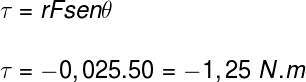Mechanics

# Torque or moment of a force

Torque , or moment of force , is the action of rotating or twisting a body around its axis of rotation (pole) through the application of a force . To apply a torque on a body, it is necessary that the force applied to it does not coincide with its axis of rotation. The distance between the point of force application and the pole is called the lever arm.

The unit of measurement for torque, according to the SI , is the newton-meter (Nm). Also, torque is a vector quantity , calculated by the cross product (or cross product) between the lever arm ( r) (also called the line of action) and the force (F). Torque and torque magnitude can be calculated using the following expression: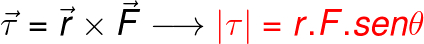Torque is the cross product of the distance from the axis of rotation and the applied force.

It is necessary to observe an important detail regarding the torque calculation: its sign. When a torque produces a counterclockwise rotation , its sign is positive, when the produced rotation happens in a clockwise direction , it is negative. In addition, it is necessary to emphasize that the torque vector is always perpendicular to the plane formed by the vectors r and F .

## What is torque?

Torque plays, for rotational movements, a role similar to that played by forces that are able to change the state of translational movements. When a non-zero resultant force is applied to a body, that body develops an acceleration , in the case of torque, in turn, its application results in the appearance of an angular acceleration. In summary, we say that torque is the dynamic agent of rotation .

According to Newton’s second law, the net force on a body is equal to its mass multiplied by the acceleration obtained by the body. Similarly, Newton’s second law for rotation states that the resultant torque on a body is equal to the product of the moment of inertia and the angular acceleration obtained by the body.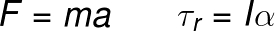τ r – resultant torque (Nm)

I – moment of inertia

α – angular acceleration

The formulas shown indicate that, in rotation, the angular acceleration obtained is inversely proportional to the moment of inertia of the body. Similarly, the acceleration obtained by a body due to the application of a force is inversely proportional to its inertia .

The moment of inertia is the resistance of bodies to change their state of rotation. Unlike inertia, the moment of inertia depends on factors such as the mass distribution and shape of a body, in addition to the position of its axis of rotation.

### Torque and Angular Moment

The classical definition of Newton ‘s second law says that the net force on a body is equal to the change in its momentum during a certain time interval. The same definition applies to torque, in this case Newton’s second law for rotation — we say that the resulting torque is equal to the change in the amount of angular momentum over a certain time. Check the similarity of these equations: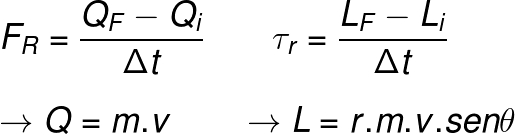Q – momentum or linear momentum

L – angular momentum

### resulting torque

When applied to bodies subject to rotational motion, Newton’s second law states that the resultant torque on a body is equal to the product of its moment of inertia by its acceleration, moreover, in all cases, it is possible to calculate the torque by the formula τ = rFsenθ , where θ is the angle between the lever arm (r) and the applied force (F).

The resulting torque is determined by the vector sum of all the torques that act on a body, moreover, in the case where the sum of the torques is zero, this body will tend to remain in rotational equilibrium : stopped or in uniform circular motion , that is, rotating with constant speed .

## Solved exercises on torque

Question 1) A force of 200 N is applied perpendicularly to the plane of a door, at a distance of 50 cm from the axis of rotation of that door, which starts to turn counterclockwise. The torque produced by this force is equal to:

a)  100.0 Nm

b) + 100.0 Nm

c) + 10,000.0 Nm

d) + 0.50 Nm

e)  2.0 Nm

Template : Letter B

Resolution:

To calculate the torque produced by the application of force, we will use the definition of torque; in addition, the distance between the pole of the door and the point of application of the force must be converted to meters; finally, since the force is applied perpendicularly to the plane of the door, the sine of angle θ will be equal to 1. Note the calculation: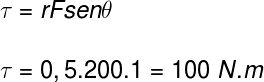The sign of this torque must be positive, since the door rotates counterclockwise, so the correct alternative is letter b.

Question 2) A circular knob, whose axis of rotation is at its center, has a diameter of 5 cm and is turned clockwise by a force of 50 N. Determine the torque applied on the knob.

a) – 1.25 Nm

b) + 1.250 Nm

c) – 1250 Nm

d) + 2500 Nm

e) – 1500 Nm

Template: letter a

Resolution :

To calculate the torque, we use the distance from the point of application of the force to the axis of rotation, which in this case is equal to the radius of the handle, 2.5 cm (0.025 m). Also, since the torque is applied clockwise, its sign is negative, note the calculation: